×
Get Full Access to CSU - Dominguez hills - MAT 119 - Class Notes - Week 8
Get Full Access to CSU - Dominguez hills - MAT 119 - Class Notes - Week 8

×

CSU - DOMINGUEZ HILLS / OTHER / MATLAB 119 / what is needed to control the output of a system accurately and reliab

# what is needed to control the output of a system accurately and reliab Description

##### Description: 1. Find the transfer function Tdy from the disturbance d to the output y (assume r = 0). Find the transfer function Try from the reference r to the output y (assume d = 0). If the transfer functions o
42 Pages 3 Views 6 Unlocks
Reviews

EEE 304 Lab Exercise 3: Linear Feedback

## what is needed to control the output of a system accurately and reliably?In order to control the output of a system accurately and reliably, a control system is required. Without a controller, the system may drift or become unstable. Take the example of a car, where your foot is on the pedal and not making adjustments. If you enter a downward slope, the car may speed up; that could be dangerous! Closing the loop with a controller on the car, exactly what a cruise control does, will help keep the speed steady. This is an example where closed-loop control can help get rid of disturbances due to unmodeled dynamics.

In another example, consider a pendulum with one end attached to a motor. In order to keep the pendulum upright many fast and accurate adjustments must be made. If a human operator attempts to do this and the pendulum rod is too short, the pendulum will fall from its upright position. A closed-loop controller with fast dynamics can however be able to stabilize the pendulum in the upright position. This is an example where an “unstable” plant is stabilized via closed loop control. What about disturbances? If you tap the pendulum gently it may even reject that disturbance and manage to stay upright!

## what is the formula for closed loop system?The above two examples serve as a brief introduction to the world of closed-loop control and why it is necessary in automation. You should get familiar with the basic concepts of linear feedback control systems from lectures, and this manual will not focus on teaching, rather on assignments. Any MATLAB command you may not have been introduced to in the previous labs will be provided. You are to complete all exercises and produce their results as asked in your reports.If you want to learn more check out What is speech?

Name:

Date:

Lab Description We also discuss several other topics like when is Medieval period?
Don't forget about the age old question of What is the Medieval Period?

Write a paragraph explaining what you have learned from this lab exercise.

For the control system shown in Fig. 1,

d

re

u

C(s) P(s) y

+ +

­

Figure 1. A closed loop control system with disturbance

where

∙ P(s) is the transfer function of the plant

## what is Target crossover frequency?∙ C(s) is the transfer function of the controller

∙ r is the reference signal

∙ e is the error signal

∙ u is the output signal from the controller

∙ d is the disturbance signal

∙ y is the output signal

1. Find the transfer function Tdy from the disturbance d to the output y (assume r =  0). Find the transfer function Try from the reference r to the output y (assume d =  0)

.

In general, the transfer function of a closed loop system is given by R(s)=G (S)

c (s)

1±G ( S) H (S )

Transfer function having d as input and y as output

Assuming r=0 , then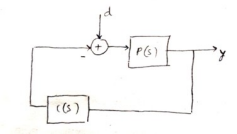d=P(s) If you want to learn more check out what is Seated Scribe?

y

1+P(s)C(s)…….(i)

Transfer function having r as input and y as output

Assuming d=0 , then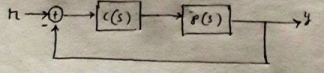G(s)=C(s) P(s), H (s)=1

y

r=C(s)P(s)

1+P(s)C(s)

2. If the transfer functions of the plant and controller are given as

∙ Plant: P(s)=1s, (e.g., a normalized description of car velocity with force as  the input; this can represent a cruise control system.)

∙ Controller: C(s)=Ks+zs, where K and z are constants.

a) Verify that this is a PI controller. Show your work and state the expression for the  P and I gains in terms of K and z.

Given controller as

C(s)=Ks+zs

C(s)=K(1+zs)

C(s)=K+kzs

Which is a proportional + an integral control

Proportional gain ¿ k

Integral gain ¿ kz

b) Show that if the input d is a step disturbance and C( 0)=∞ , the effect of d on y approaches zero as t → ∞ and (Hint: Assume K,z > 0. Use the final value theorem.) Demonstrate your result by choosing the following different sets of values for K and z (varied by orders of magnitude) and plotting their closed-loop step response. Include MATLAB code and plots. Discuss your observation.

From equation (i) Don't forget about the age old question of what is Circular flow of income?

y (s)=P(s)

1+P(s)C (s).d (s)

When d(s) is step input

1

1+1s. ks+zs.1s

y (s)=

s

y (t →∞)=lim s=0

1

sy (s)

¿ lim s=0

s

1+k (s+z) s2

=0

1) K = 0.1, z = 0.1

A= tf([1 0] , [1 0.1 0.1]);   % Tdy with k=0.1 , z=0.1 subplot(311), step(A)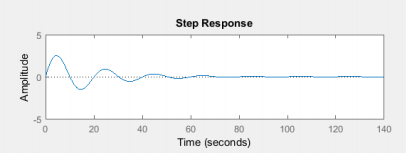2) K = 1, z = 1

B= tf([1 0] , [1 1 1]);       % Tdy with k=1 , z=1

subplot(312), step(B)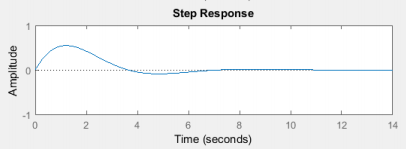3) K = 10, z = 10

C= tf([1 0] , [1 10 10]);     % Tdy with k=10 , z=10

subplot(313), step(C)

4)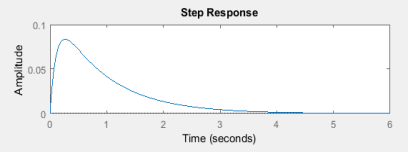We also discuss several other topics like What are the Basic characteristics of living organisms?

c) Design a controller (select values of K and z) such that the following specifications are met:

∙ Target crossover frequency = 1 rad/s (approximately equal to the closed-loop bandwidth).

∙ Target phase margin is 60⁰.

1) Show all your work and demonstrate your design meets these specifications by the use of “allmargin” command on the open-loop transfer function L(s) = P(s)C(s). (hint: “help allmargin” in Matlab). Include MATLAB code and results.

The transfer function is

r=C (s) P (s)

y

1+C(s) P(s)

y

r=

k (s+z)

z∗1

s

k (s+z)

z∗1

s )

y

1+(

r=ks+kz

s2+ks+kz

Replacing  s= jw

w

j ¿

r=( kz−kjw)

y

(¿¿ 2+kjw+kz)

r=(kz−kjw)

y

( kz−w2+kjw)

At crossover frequency |KG(s) = 1|

Equating the magnitude of above transfer equation to unity; √( kz)2+(kw)2

( kz−w2)+( kw)2=1

( kz)2+( k )2

( kz−12)+( k )2=1

(kz)2+k2=( kz)2−2 kz+1+(k )2

0=−2 kz+1

kz=12

Phase margin;

tan−1 kwkz−tan−1 kw

kz−w2=180−60

tan−12 k−tan−1(−2 k )=180−60

2 tan−12 k=120

1

2tan 60=k , k=0.866

Result from the all margin command

S =

GainMargin: 0

GMFrequency: 0

PhaseMargin: 59.9989

PMFrequency: 1.0000

DelayMargin: 1.0472

DMFrequency: 1.0000

Stable: 1

2) Plot the closed-loop frequency and step responses from the reference input r to the output y. Show the achieved bandwidth by marking on the Bode plot with a “data cursor”. Comment on how different, if so, it is from the specified bandwidth. Include MATLAB code and plots. (Read http://www.mathworks.com/help/matlab/creating_plots/data-cursor-displaying

data-values-interactively.html on how to use the data cursor.)

y

r=C(s)P(s)

1+P(s)C(s)

y

r=ks+kz

s2+ks+kz

y

r=0.866 s+0.5

s2+0,866 s+0.5

Step response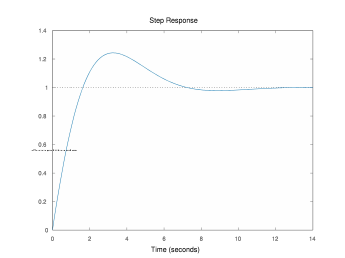Frequency response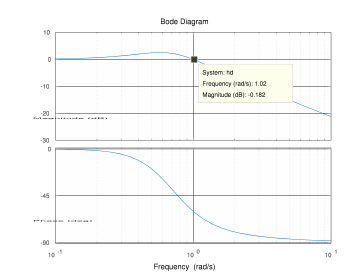clc

clear

% Transfer function

hd = tf([0.866 0.5], [1 0.866 0.5]);

% step responce

figure(1)

step(hd)

% bode plot

figure(2)

bode(hd)

grid on

3) Plot the closed-loop frequency and step responses from the disturbance d to the output y. Does the steady state error e become 0 when a step disturbance d is applied to this closed loop transfer function? Include MATLAB code and plots.

Transfer function of disturbance

d=P(s)

y

1+P(s)C(s)

d=s

y

s2+ks+kz

d=s

y

s2+0.866 s+0.5

Step response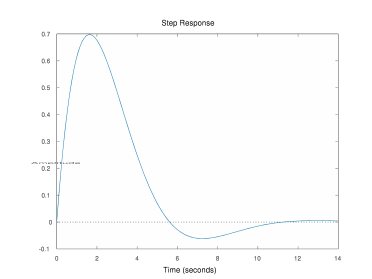Frequency response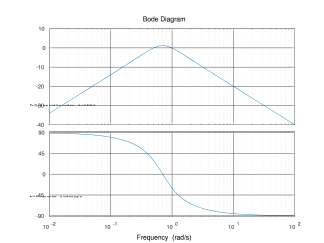3. Now design a proportional-only controller (i.e., C(s) = K) to have the same closed loop crossover frequency (bandwidth) = 1 rad/s. Show your work. You can ignore the phase margin requirement, since it cannot be modified without a zero in the controller. Repeat problem 2 c) part 2) and 3). Is an integrator needed in the controller? Explain your observation.

For input r output y

y

r=C(s)P(s)

1+P(s)C(s)

But C(s) = k

y

r=k

s+k

y

r=0.866

s+0.866

Step response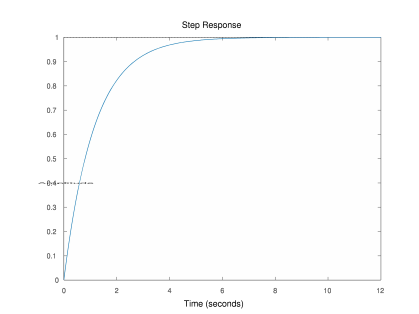Frequency response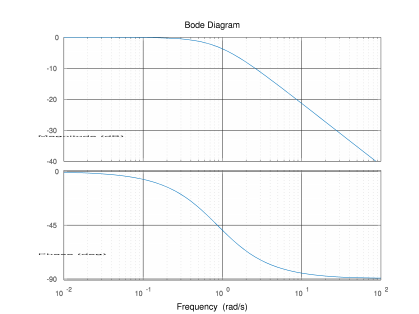For input d output y

d=P(s)

y

1+P(s)C(s)

d=1s+k

y

d=1

y

s+0.866

Step response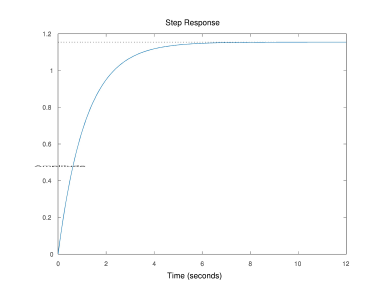Frequency response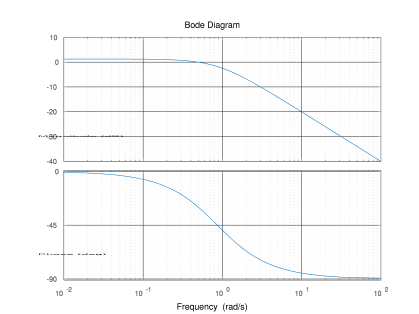NOTE: in order to form a closed loop transfer function you need to utilize the  “feedback” command. Assuming you have created transfer function objects for both  the plant and controller, i.e., P and C, the closed-loop transfer function from r to y, Try, will be computed as

>> T_ry = feedback(P*C,1)

Subsequently the bode plot and step response for Try can be found with

>> bode(T_ry)

>> step(T_ry)

In order to compute the transfer function from the disturbance d to the output y, use >> T_dy = feedback(P, C)

This problem explores the effect of sensor noise on the input to the plant. Plant inputs are typically provided by actuators, e.g., valves, motors, and other mechanical components. High-frequency inputs to these actuators cause more stress, leading to shorter actuator lifetime and higher probability of actuator failure. The sensors that measure the output and feed it back to the controller are prone to high-frequency measurement noise. This problem will show the trade-off between bandwidth and high-frequency noise attenuation. Fig. 2 shows how sensor noise enters into the closed-loop system as the signal n(t):

re

­

u

C(s) P(s) y

n

Figure 2. A closed loop control system with noise

1. Given the plant P(s)=1s, design a PI controller C(s)=Ks+zs as in Task 1,  problem 2, part c), same phase margin but for a target crossover frequency of 10  rad/s. Repeat Task 1, problem 2, part c) 1) and 2).

Part c 1

The transfer function is given by; where

c s+

k s z

( )

=

( ) z

p s1

=

( )s

y

r=C (s) P (s)

y

1+C(s) P(s)

k (s+z)

z∗1

s

r=

k (s+z)

z∗1

s )

y

1+(

r=ks+kz

s2+ks+kz

Replacing s with jw;

kz kj

+

2

ω

=ω ω

kz kj

− +

At crossover frequency |KG(s) = 1|

Equating the magnitude of the transfer function to  2 2

kz k

+

ω

( ) ( )

( ) ( )1 2 2

=

kz k − +

ω ω

=

2 2 2 2

kz +100k = kz −100 +100k

( ) ( )

2 2 2 2

kz +100k = kz − 200kz +10000 +100k

( ) ( )

200kz =10000

kz = 50

The phase of the transfer is obtained as;

−  k − k

10

10

−

tan 1 1 = −



50

 −

tan

50 100

180 60

10 2 tan 1 =

−  k

120



50

10k

=2120

Thus z and k is obtained as

50

tan





50 k = =

10

tan( 60) 8.66 50

S =

z = = 8.66

5.774

GainMargin: 0

GMFrequency: 0

PhaseMargin: 59.9989

PMFrequency: 9.9998

DelayMargin: 0.1047

DMFrequency: 9.9998

Stable: 1

Matlab code

clc

clear

% Transfer function

hd = tf([8.66 50], [1 0 0]);

% Gain margin, Phase margin,

S = allmargin(hd)

Part c 2

Frequency response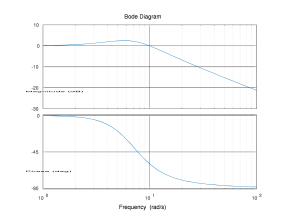Step responce2. Compare the closed-loop frequency responses from the sensor noise n to the  plant input u (Tnu = feedback(C, P)) for this controller and the controller you  designed in Task 1, problem 2, part c) with crossover frequency 1. Comment on  the difference and what you think is happening. Include MATLAB code and plots.

Tnu = feedback(C, P)

Frequency response

Matlab code

clc

clear

% Transfer function

C = tf(,[1 0]);

P = tf([0.886 0.5],[1 0]);

hd = feedback(C,P);

% bode plot

figure(1)

bode(hd)

grid on

3. In order to visualize the effects, you will now simulate the two closed loop responses in Simulink. At this point, we assume that you have transfer functions for both controllers. Create a Simulink model as shown below:

In the top loop, use the controller with crossover frequency 1 and the bottom loop use the controller with crossover frequency 10. Use the following Simulink blocks:  ∙ Sum (under Simulink->Commonly Used Blocks): “List of Signs”, “|+-“ for +- block and “|++“ for ++ block

∙ Transfer function (under Simulink->Continuous): specify numerator and denominator coefficients

∙ Band-limited white noise (under Simulink->Sources): noise power = 0.0001

∙ Scope (under Simulink->Sinks): double click open scope, click parameters (next to the printer icon), change “number of axes” to 2. Now it can display two graphs.

Run the simulation and provide plots of the output. Justify your answer to problem 2 using these step responses.

This problem studies the effect of unmodeled lag on closed-loop system behavior. The following transfer function represents a perturbation to the system in Fig. 1.  ∙ Plant: P(s)=1

s(as+1)

The term 1/as+1 can be viewed as a 1st order lag introduced by unmodeled actuator dynamics (engine; e.g., throttle to torque/force response) or sensor dynamics. The objective of this problem is to demonstrate that when such lags alter the system significantly around the crossover frequency, the closed-loop may become unstable and the controller must be redesigned. Notice that the achievable performance may no longer be the same.

1. Consider the cases where a = 0, 0.1, 1, 10 and plot the closed-loop frequency and step responses from r to y for the same controller designed in Task 1, problem 2,  part c). (Note: The case of a = 0 is the nominal plant with no lag.) Include MATLAB code and plots.

Frequency response

Matlab code

clc

clear

% Transfer function

C = tf(,[1 0]);

a=0;

P = tf([1 0],[a 1 0]);

hd0 = feedback(C*P,1);

a=0.1;

P = tf([1 0],[a 1 0]);

hd1 = feedback(C*P,1);

a=1;

P = tf([1 0],[a 1 0]);

hd2 = feedback(C*P,1);

a=10;

P = tf([1 0],[a 1 0]);

hd3 = feedback(C*P,1);

% step responce

figure(1)

step(hd0)

hold on

step(hd1)

hold on

step(hd2)

hold on

step(hd3)

hold off

% bode plot

figure(2)

bode(hd0)

hold on

bode(hd1)

hold on

bode(hd2)

hold on

bode(hd3)

hold off

grid on

2. For the case a = 10, redesign the controller to achieve 60o phase margin and  crossover frequency of 0.036 rad/s. Demonstrate your design meets these  specifications by the use of “allmargin” command on L(s) = P(s)C(s). Plot the  closed-loop frequency and step responses from r to y. Include MATLAB code and  plots.

As you see, you were able to stabilize the controller but at the cost of bandwidth. The system is stable but sluggish now. You do not need to perform any further experiment but know that the sacrifice in speed would not have been necessary if a PID controller is used in this case rather than a PI.

LAB 3: LAB REPORT GRADE SHEET

Name

Instructor Assessment

Grading Criteria Max Points Points Lost Template

Neatness, Clarity, and Concision

Lab Description

Description of Assigned Tasks, Work Performed  & Outcomes Met

Lab Score (out of 50)

2

8

15

15

10

Points

Lost Late Lab

Lab

Score

EEE 304 Lab Exercise 3: Linear Feedback

In order to control the output of a system accurately and reliably, a control system is required. Without a controller, the system may drift or become unstable. Take the example of a car, where your foot is on the pedal and not making adjustments. If you enter a downward slope, the car may speed up; that could be dangerous! Closing the loop with a controller on the car, exactly what a cruise control does, will help keep the speed steady. This is an example where closed-loop control can help get rid of disturbances due to unmodeled dynamics.

In another example, consider a pendulum with one end attached to a motor. In order to keep the pendulum upright many fast and accurate adjustments must be made. If a human operator attempts to do this and the pendulum rod is too short, the pendulum will fall from its upright position. A closed-loop controller with fast dynamics can however be able to stabilize the pendulum in the upright position. This is an example where an “unstable” plant is stabilized via closed loop control. What about disturbances? If you tap the pendulum gently it may even reject that disturbance and manage to stay upright!

The above two examples serve as a brief introduction to the world of closed-loop control and why it is necessary in automation. You should get familiar with the basic concepts of linear feedback control systems from lectures, and this manual will not focus on teaching, rather on assignments. Any MATLAB command you may not have been introduced to in the previous labs will be provided. You are to complete all exercises and produce their results as asked in your reports.

Name:

Date:

Lab Description

Write a paragraph explaining what you have learned from this lab exercise.

For the control system shown in Fig. 1,

d

re

u

C(s) P(s) y

+ +

­

Figure 1. A closed loop control system with disturbance

where

∙ P(s) is the transfer function of the plant

∙ C(s) is the transfer function of the controller

∙ r is the reference signal

∙ e is the error signal

∙ u is the output signal from the controller

∙ d is the disturbance signal

∙ y is the output signal

1. Find the transfer function Tdy from the disturbance d to the output y (assume r =  0). Find the transfer function Try from the reference r to the output y (assume d =  0)

.

In general, the transfer function of a closed loop system is given by R(s)=G (S)

c (s)

1±G ( S) H (S )

Transfer function having d as input and y as output

Assuming r=0 , then

d=P(s)

y

1+P(s)C(s)…….(i)

Transfer function having r as input and y as output

Assuming d=0 , then

G(s)=C(s) P(s), H (s)=1

y

r=C(s)P(s)

1+P(s)C(s)

2. If the transfer functions of the plant and controller are given as

∙ Plant: P(s)=1s, (e.g., a normalized description of car velocity with force as  the input; this can represent a cruise control system.)

∙ Controller: C(s)=Ks+zs, where K and z are constants.

a) Verify that this is a PI controller. Show your work and state the expression for the  P and I gains in terms of K and z.

Given controller as

C(s)=Ks+zs

C(s)=K(1+zs)

C(s)=K+kzs

Which is a proportional + an integral control

Proportional gain ¿ k

Integral gain ¿ kz

b) Show that if the input d is a step disturbance and C( 0)=∞ , the effect of d on y approaches zero as t → ∞ and (Hint: Assume K,z > 0. Use the final value theorem.) Demonstrate your result by choosing the following different sets of values for K and z (varied by orders of magnitude) and plotting their closed-loop step response. Include MATLAB code and plots. Discuss your observation.

From equation (i)

y (s)=P(s)

1+P(s)C (s).d (s)

When d(s) is step input

1

1+1s. ks+zs.1s

y (s)=

s

y (t →∞)=lim s=0

1

sy (s)

¿ lim s=0

s

1+k (s+z) s2

=0

1) K = 0.1, z = 0.1

A= tf([1 0] , [1 0.1 0.1]);   % Tdy with k=0.1 , z=0.1 subplot(311), step(A)

2) K = 1, z = 1

B= tf([1 0] , [1 1 1]);       % Tdy with k=1 , z=1

subplot(312), step(B)

3) K = 10, z = 10

C= tf([1 0] , [1 10 10]);     % Tdy with k=10 , z=10

subplot(313), step(C)

4)

c) Design a controller (select values of K and z) such that the following specifications are met:

∙ Target crossover frequency = 1 rad/s (approximately equal to the closed-loop bandwidth).

∙ Target phase margin is 60⁰.

1) Show all your work and demonstrate your design meets these specifications by the use of “allmargin” command on the open-loop transfer function L(s) = P(s)C(s). (hint: “help allmargin” in Matlab). Include MATLAB code and results.

The transfer function is

r=C (s) P (s)

y

1+C(s) P(s)

y

r=

k (s+z)

z∗1

s

k (s+z)

z∗1

s )

y

1+(

r=ks+kz

s2+ks+kz

Replacing  s= jw

w

j ¿

r=( kz−kjw)

y

(¿¿ 2+kjw+kz)

r=(kz−kjw)

y

( kz−w2+kjw)

At crossover frequency |KG(s) = 1|

Equating the magnitude of above transfer equation to unity; √( kz)2+(kw)2

( kz−w2)+( kw)2=1

( kz)2+( k )2

( kz−12)+( k )2=1

(kz)2+k2=( kz)2−2 kz+1+(k )2

0=−2 kz+1

kz=12

Phase margin;

tan−1 kwkz−tan−1 kw

kz−w2=180−60

tan−12 k−tan−1(−2 k )=180−60

2 tan−12 k=120

1

2tan 60=k , k=0.866

Result from the all margin command

S =

GainMargin: 0

GMFrequency: 0

PhaseMargin: 59.9989

PMFrequency: 1.0000

DelayMargin: 1.0472

DMFrequency: 1.0000

Stable: 1

2) Plot the closed-loop frequency and step responses from the reference input r to the output y. Show the achieved bandwidth by marking on the Bode plot with a “data cursor”. Comment on how different, if so, it is from the specified bandwidth. Include MATLAB code and plots. (Read http://www.mathworks.com/help/matlab/creating_plots/data-cursor-displaying

data-values-interactively.html on how to use the data cursor.)

y

r=C(s)P(s)

1+P(s)C(s)

y

r=ks+kz

s2+ks+kz

y

r=0.866 s+0.5

s2+0,866 s+0.5

Step response

Frequency response

clc

clear

% Transfer function

hd = tf([0.866 0.5], [1 0.866 0.5]);

% step responce

figure(1)

step(hd)

% bode plot

figure(2)

bode(hd)

grid on

3) Plot the closed-loop frequency and step responses from the disturbance d to the output y. Does the steady state error e become 0 when a step disturbance d is applied to this closed loop transfer function? Include MATLAB code and plots.

Transfer function of disturbance

d=P(s)

y

1+P(s)C(s)

d=s

y

s2+ks+kz

d=s

y

s2+0.866 s+0.5

Step response

Frequency response

3. Now design a proportional-only controller (i.e., C(s) = K) to have the same closed loop crossover frequency (bandwidth) = 1 rad/s. Show your work. You can ignore the phase margin requirement, since it cannot be modified without a zero in the controller. Repeat problem 2 c) part 2) and 3). Is an integrator needed in the controller? Explain your observation.

For input r output y

y

r=C(s)P(s)

1+P(s)C(s)

But C(s) = k

y

r=k

s+k

y

r=0.866

s+0.866

Step response

Frequency response

For input d output y

d=P(s)

y

1+P(s)C(s)

d=1s+k

y

d=1

y

s+0.866

Step response

Frequency response

NOTE: in order to form a closed loop transfer function you need to utilize the  “feedback” command. Assuming you have created transfer function objects for both  the plant and controller, i.e., P and C, the closed-loop transfer function from r to y, Try, will be computed as

>> T_ry = feedback(P*C,1)

Subsequently the bode plot and step response for Try can be found with

>> bode(T_ry)

>> step(T_ry)

In order to compute the transfer function from the disturbance d to the output y, use >> T_dy = feedback(P, C)

This problem explores the effect of sensor noise on the input to the plant. Plant inputs are typically provided by actuators, e.g., valves, motors, and other mechanical components. High-frequency inputs to these actuators cause more stress, leading to shorter actuator lifetime and higher probability of actuator failure. The sensors that measure the output and feed it back to the controller are prone to high-frequency measurement noise. This problem will show the trade-off between bandwidth and high-frequency noise attenuation. Fig. 2 shows how sensor noise enters into the closed-loop system as the signal n(t):

re

­

u

C(s) P(s) y

n

Figure 2. A closed loop control system with noise

1. Given the plant P(s)=1s, design a PI controller C(s)=Ks+zs as in Task 1,  problem 2, part c), same phase margin but for a target crossover frequency of 10  rad/s. Repeat Task 1, problem 2, part c) 1) and 2).

Part c 1

The transfer function is given by; where

c s+

k s z

( )

=

( ) z

p s1

=

( )s

y

r=C (s) P (s)

y

1+C(s) P(s)

k (s+z)

z∗1

s

r=

k (s+z)

z∗1

s )

y

1+(

r=ks+kz

s2+ks+kz

Replacing s with jw;

kz kj

+

2

ω

=ω ω

kz kj

− +

At crossover frequency |KG(s) = 1|

Equating the magnitude of the transfer function to  2 2

kz k

+

ω

( ) ( )

( ) ( )1 2 2

=

kz k − +

ω ω

=

2 2 2 2

kz +100k = kz −100 +100k

( ) ( )

2 2 2 2

kz +100k = kz − 200kz +10000 +100k

( ) ( )

200kz =10000

kz = 50

The phase of the transfer is obtained as;

−  k − k

10

10

−

tan 1 1 = −



50

 −

tan

50 100

180 60

10 2 tan 1 =

−  k

120



50

10k

=2120

Thus z and k is obtained as

50

tan





50 k = =

10

tan( 60) 8.66 50

S =

z = = 8.66

5.774

GainMargin: 0

GMFrequency: 0

PhaseMargin: 59.9989

PMFrequency: 9.9998

DelayMargin: 0.1047

DMFrequency: 9.9998

Stable: 1

Matlab code

clc

clear

% Transfer function

hd = tf([8.66 50], [1 0 0]);

% Gain margin, Phase margin,

S = allmargin(hd)

Part c 2

Frequency response

Step responce

2. Compare the closed-loop frequency responses from the sensor noise n to the  plant input u (Tnu = feedback(C, P)) for this controller and the controller you  designed in Task 1, problem 2, part c) with crossover frequency 1. Comment on  the difference and what you think is happening. Include MATLAB code and plots.

Tnu = feedback(C, P)

Frequency response

Matlab code

clc

clear

% Transfer function

C = tf(,[1 0]);

P = tf([0.886 0.5],[1 0]);

hd = feedback(C,P);

% bode plot

figure(1)

bode(hd)

grid on

3. In order to visualize the effects, you will now simulate the two closed loop responses in Simulink. At this point, we assume that you have transfer functions for both controllers. Create a Simulink model as shown below:

In the top loop, use the controller with crossover frequency 1 and the bottom loop use the controller with crossover frequency 10. Use the following Simulink blocks:  ∙ Sum (under Simulink->Commonly Used Blocks): “List of Signs”, “|+-“ for +- block and “|++“ for ++ block

∙ Transfer function (under Simulink->Continuous): specify numerator and denominator coefficients

∙ Band-limited white noise (under Simulink->Sources): noise power = 0.0001

∙ Scope (under Simulink->Sinks): double click open scope, click parameters (next to the printer icon), change “number of axes” to 2. Now it can display two graphs.

Run the simulation and provide plots of the output. Justify your answer to problem 2 using these step responses.

This problem studies the effect of unmodeled lag on closed-loop system behavior. The following transfer function represents a perturbation to the system in Fig. 1.  ∙ Plant: P(s)=1

s(as+1)

The term 1/as+1 can be viewed as a 1st order lag introduced by unmodeled actuator dynamics (engine; e.g., throttle to torque/force response) or sensor dynamics. The objective of this problem is to demonstrate that when such lags alter the system significantly around the crossover frequency, the closed-loop may become unstable and the controller must be redesigned. Notice that the achievable performance may no longer be the same.

1. Consider the cases where a = 0, 0.1, 1, 10 and plot the closed-loop frequency and step responses from r to y for the same controller designed in Task 1, problem 2,  part c). (Note: The case of a = 0 is the nominal plant with no lag.) Include MATLAB code and plots.

Frequency response

Matlab code

clc

clear

% Transfer function

C = tf(,[1 0]);

a=0;

P = tf([1 0],[a 1 0]);

hd0 = feedback(C*P,1);

a=0.1;

P = tf([1 0],[a 1 0]);

hd1 = feedback(C*P,1);

a=1;

P = tf([1 0],[a 1 0]);

hd2 = feedback(C*P,1);

a=10;

P = tf([1 0],[a 1 0]);

hd3 = feedback(C*P,1);

% step responce

figure(1)

step(hd0)

hold on

step(hd1)

hold on

step(hd2)

hold on

step(hd3)

hold off

% bode plot

figure(2)

bode(hd0)

hold on

bode(hd1)

hold on

bode(hd2)

hold on

bode(hd3)

hold off

grid on

2. For the case a = 10, redesign the controller to achieve 60o phase margin and  crossover frequency of 0.036 rad/s. Demonstrate your design meets these  specifications by the use of “allmargin” command on L(s) = P(s)C(s). Plot the  closed-loop frequency and step responses from r to y. Include MATLAB code and  plots.

As you see, you were able to stabilize the controller but at the cost of bandwidth. The system is stable but sluggish now. You do not need to perform any further experiment but know that the sacrifice in speed would not have been necessary if a PID controller is used in this case rather than a PI.

LAB 3: LAB REPORT GRADE SHEET

Name

Instructor Assessment

Grading Criteria Max Points Points Lost Template

Neatness, Clarity, and Concision

Lab Description

Description of Assigned Tasks, Work Performed  & Outcomes Met

Lab Score (out of 50)

2

8

15

15

10

Points

Lost Late Lab

Lab

Score

Page ExpiredIt looks like your free minutes have expired! Lucky for you we have all the content you need, just sign up here